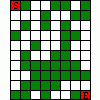2018-7-12 00:46
2108

## 代码分析### 主流程分析### 计算函数分析## check分析

0 < s < 10

```sqrt(s1**2+s2**2+s3**2) > 15.5

s1**2+s2**2+s3**2 > 240.25 >= 241

240 /3 = 80
9*9 = 81
8*8 = 64

```

abs(num1+num2-s) < 0.003

```1. 输入长度为10，且字符范围是数字+A-F
2. 1<num1<10; 1<num2<10
3. num1+num2 == 10 (假定)
4. num1+num2-s < 0.003
5. input == '0'
```

## 求解```1 < num1 < 1.125
8 < num1 < 8.2
```

```import numpy as np
import struct
import os
import matplotlib.pyplot as plt

step = 0.00005

a = [ -1.0245,    0.0848024,
-0.530959,  0.703843,
-0.120586,  -0.313185,
-0.765289,  0.339469,
0.40869,    0.346429,
-0.672084,  0.703138,
0.479381,   0.145042,
-0.758163,  -1.19117,
-0.716324,  -0.185696,
-0.388104,  -0.441494,
0.749153,   -0.293723,
-0.280767,  -0.660177,
-1.13947,   -0.373301,
-0.371646,  -0.320964,
0.227951,   0.286258,
-0.64833,   0.34251,
0.101866,   -0.0669482,
-0.799297,  0.277074]
a_1 = np.array([-1.0245, -0.530959, -0.120586, -0.765289, 0.40869, -0.672084,
0.479381, -0.758163, -0.716324, -0.388104, 0.749153, -0.280767,
-1.13947, -0.371646, 0.227951, -0.64833, 0.101866, -0.799297])
a_2 = np.array([0.0848024, 0.703843, -0.313185, 0.339469, 0.346429, 0.703138,
0.145042, -1.19117, -0.185696, -0.441494, -0.293723, -0.660177,
-0.373301, -0.320964, 0.286258, 0.34251, -0.0669482, 0.277074])
w = [-0.119411,0.364227,-0.335042,-0.616251,1.03833,0.193202,
1.00183,0.443425,-0.571216,-1.36825,0.158766,-1.15323,
0.253585,-0.988879,0.611415,0.149166,0.518852,0.298557]

#1.0625   8.9375
##flag:F13FE02140
def crack():
i1 = 1
while True:
i1 = i1+step
#  i1 = 1.0625
i2 = 10-i1
x = (i1-0.01+1)/(9.99-0.01+1)
y = (i2-0.03+1)/(9.99-0.03+1)
l = []

for i in range(18):
t1 = x*a[2*i]
t2 = y*a[2*i+1]
l.append(1.0/(np.exp(-1*(t1+t2))+1))
s = 0
ss = 0
for i in range(18):
s += l[i]*w[i]
ss = s*(19.32-0.26+1)+0.26-1

if ss<10 and ss>9.997 :
d1 = struct.pack('>d',round(i1,6))
d2 = struct.pack('>d',round(i2,6))
if d1[2:] == '\x00'*6 and d2[3:] == '\x00'*5:
print i1,d1.encode('hex').upper()
print i2,d2.encode('hex').upper()
print ss
if i1 >1.2:
return

def dataplot(r1,r2,step):
a1 = a_1.reshape(18,1)
a2 = a_2.reshape(18,1)
wi = np.array(w)
X=np.arange(r1,r2,step)
Y = 10-X
x = (X-0.01+1)/(9.99-0.01+1)
y = (Y-0.03+1)/(9.99-0.03+1)
t1 = x*a1
t2 = y*a2
l = 1.0/(np.exp(-1*(t1+t2))+1)
s = np.dot(l.T,wi)
ss = s*(19.32-0.26+1)+0.26-1
plt.plot(X,ss)

plt.figure(1)
plt.subplot(111)
plt.grid()
dataplot(1,9,0.0005)
plt.figure(2)
plt.subplot(211)
plt.grid()
dataplot(1,1.2,0.0005)
plt.subplot(212)
plt.grid()
dataplot(8,8.6,0.005)
plt.show()
crack()
```

【公告】看雪招聘大学实习生！看雪20年安全圈的口碑，助你快速成长！lelfei23 2018-7-12 18:21
2
0poyoten22 2018-7-12 19:28
3
0lelfei 这个牛，基本分析出神经网络的结构了，赞一个lelfei23 2018-7-12 21:14
4
0poyoten 还不是猜错结构了[em_5]poyoten22 2018-7-12 21:28
5
0lelfei 你的解法是最接近神经网络计算过程的，其他人都太暴力了[em_2]